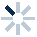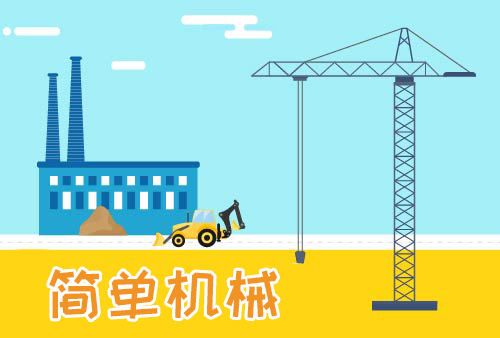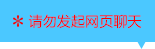| | |

||
APP端下载AndroidiPhone
￥54

|
1人点赞
3478人已学习
|

3天无理由退款

3天无理由退款：退款将以超级币形式退至您的超级课堂学习账户，便于您重新选购其他课程。恶意退款将被冻结账号。

• 1、介绍了液体的压强变化量公式，$\Delta p=ρ_{液}g\Delta h$
2、 学习液体压强的一种等效理解方式
3、 无论是下压还是上提漂浮物，压强变化量还是要用$\Delta p=ρ_{液}g\Delta h$去算
• 1、若柱形容器底面积为$S$，物体浸入液体的体积为$V_{排}$时，液体对容器底部的压强的增量为$\Delta p=ρ_{液}g\Delta h=\dfrac{ρ_{液}gV_{排}}{S}$，压力的增量为$\Delta F=ρ_{液}gV_{排}$
2、 这两个结论，能够帮助我们解决涉及浸入物体后，容器底部受到的压强压力变化的题目
• 1、我们学到的第一个结论，是往底面积均为$S$的三种容器中浸入相同体积的物体后，锥形容器的压强增量较大，盆形容器的压强增量较小
2、 第二个结论，是关于漂浮物或悬浮物使容器底部受到的液体压力增量
3、 在最后一题的第四问中，要注意把木块和压它的铁丝，等效转化成悬浮物。从而通过比较液体密度，来比较悬浮物的质量
• 1、利用体积恒等关系$V_{总}=V_{0}+V_{浸}$，帮助我们研究$h_{0}$，$h_{浸}$，$h_{液}$这三个量之间的关系，或者$h_{液}$和$V_{0}$之间的关系
• 1、当直柱形物体浸入直柱形容器中的液体并上下移动时，存在比例式$\dfrac{\Delta h_{液}}{\Delta h_{物}}=\dfrac{S_{物}}{S_{器}-S_{物}}$
2、 物体浸入深度的变化量，是由液面上升量和物体下沉量相加产生的，即$\Delta h_{液}+\Delta h_{物}=\Delta h_{浸}$
3、 反过来，如果把物块上提，液面下降，依旧满足该比例式和加法式
4、 要注意的是，以上公式和规律有三个前提，一是物体和容器都要是直柱形，否则推导公式时用的体积公式就不成立；二是物体没有全部浸入液体，否则液面高度不会有变化；三是液体未溢出，否则不满足体积相等
• 1、介绍两类特殊的物浸液动态问题：第一类题型，是物体在上下移动的过程中，经历过全部在水面上，或全部在水面下的阶段。这两个阶段，是不会导致水面高度变化的。所以要把它们和入水，出水阶段分开研究
2、 第二类题型，是物体不满足单一直柱体，而是由多个横截面积不同，即$S$物不同的直柱体组合而成。此时，可以根据$S$物的不同来分类计算
3、 在题目中还要注意检验物体是否完全浸没，水是否会溢出等因素，这样才能合理使用上节课介绍的两个公式
• 1、介绍盛有液体的容器的支持面所受的压力和压强：对于直柱形容器，“支持面受到的压力$F$”，等于“液体对容器底的压力$F_{液}+$容器的重力$F_{器}$”；对于物浸液问题，可以用等效法思考，就能回归刚才的方法了
2、 在具体题目中，要熟练应用之前学过的体积恒等关系式，以及物浸液的压强增量公式
• 1、物浸液问题相关知识点的整体回顾
2、 练习一道复杂的综合问题

• 11441350576
• 2超级学员458552
• 3超级学员850181
• 4超级学员1838498
• 5wdnmd
• 6超级学员2093670
• 7超级学员2367054
• 8超级学员2692318164493人在学
￥ 100 ￥ 78163101人在学
￥ 40 ￥ 32164607人在学
￥ 80 ￥ 64163890人在学
￥ 40 ￥ 32

• 0
• 点击分享有好礼
••app端下载关注微信号